# RD Sharma Solutions For Class 7 Maths Chapter 14 Lines And Angles Exercise 14.1

RD Sharma Solutions for Class 7 Maths Exercise 14.1 of Chapter 14 Lines And Angles in PDF are available here. Students can refer and download it from the available links. This exercise has thirty-two questions. RD Sharma Solutions for Class 7 provides solutions for all topics covered in this chapter. Exercise 14.1 mainly deals with a pair of angles and their properties. Let us have a look at some of the important topics present in this exercise.

• Pairs of Angles
• Definition and meaning of adjacent angles
• Definition and meaning of linear pair
• Vertically opposite angles
• Definition of angles at a point
• Concept of complementary angles
• Concept of supplementary angles

## Download the PDF of RD Sharma Solutions For Class 7 Maths Chapter 14 – Lines And Angles Exercise 14.1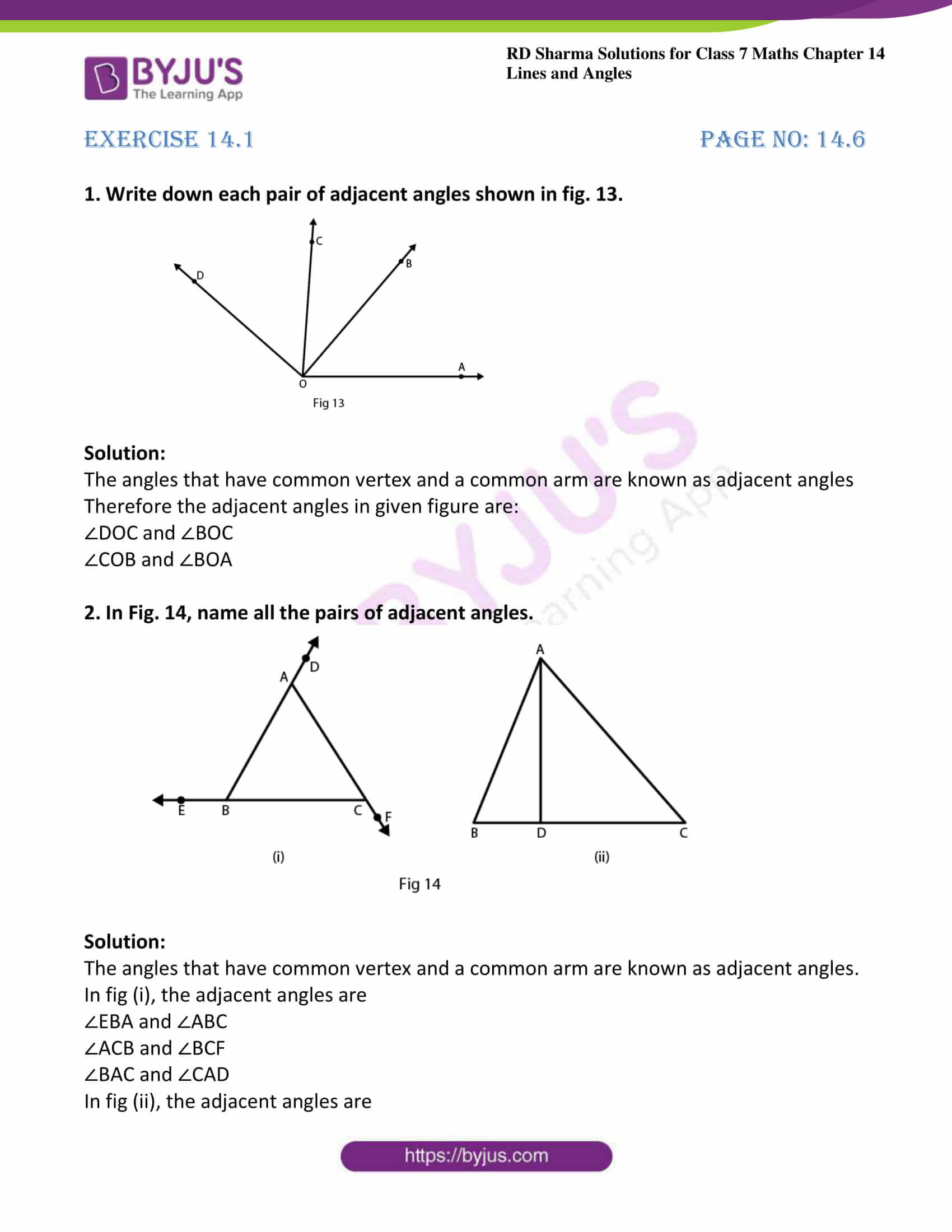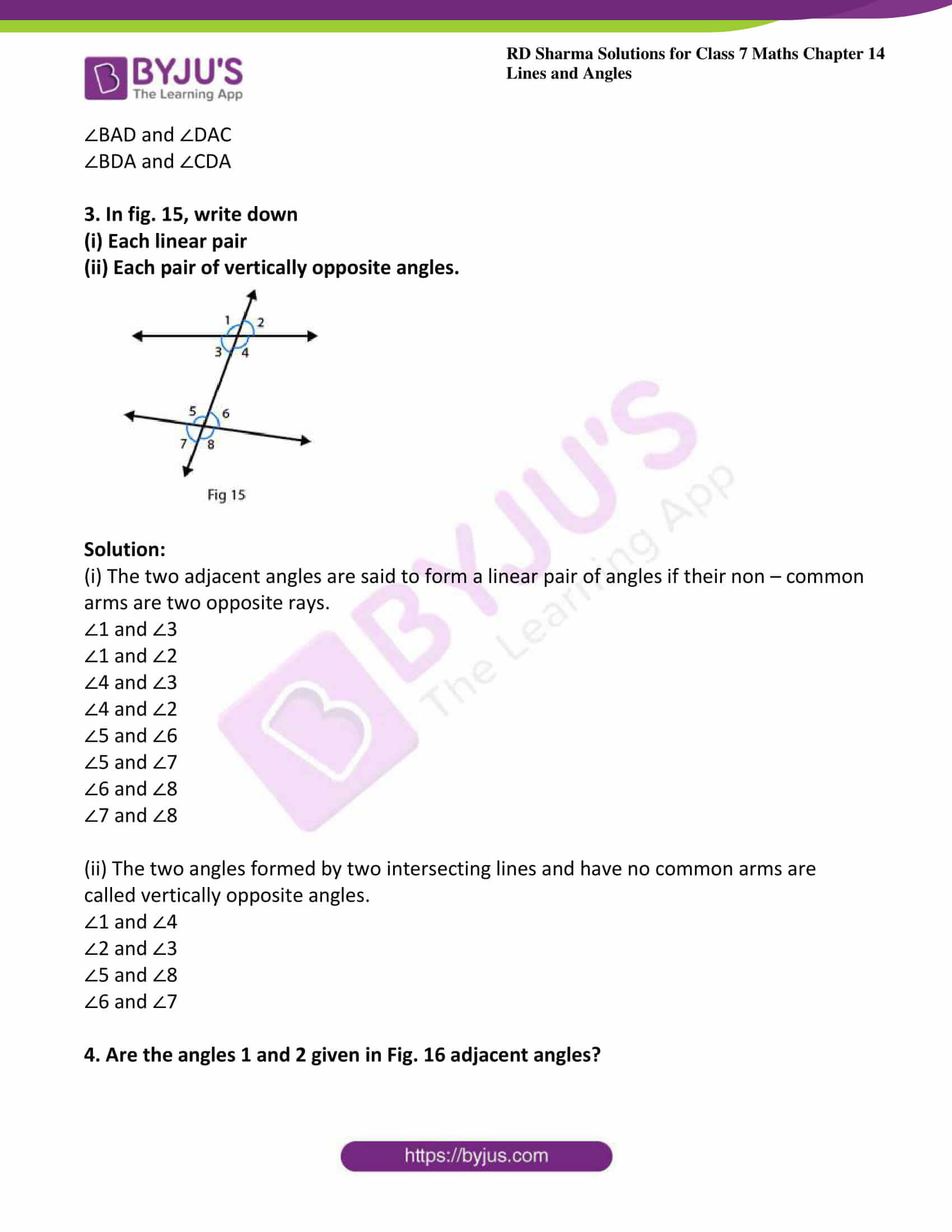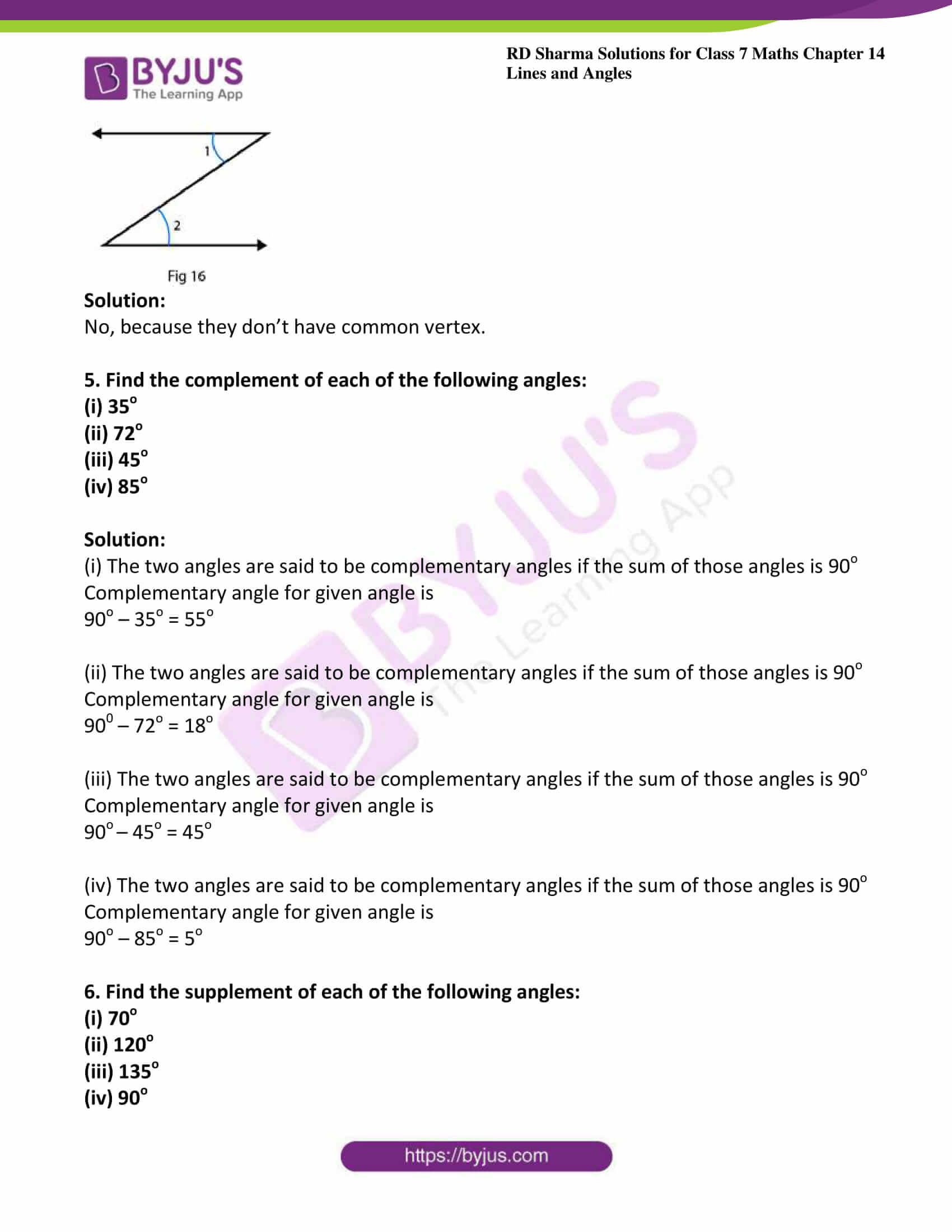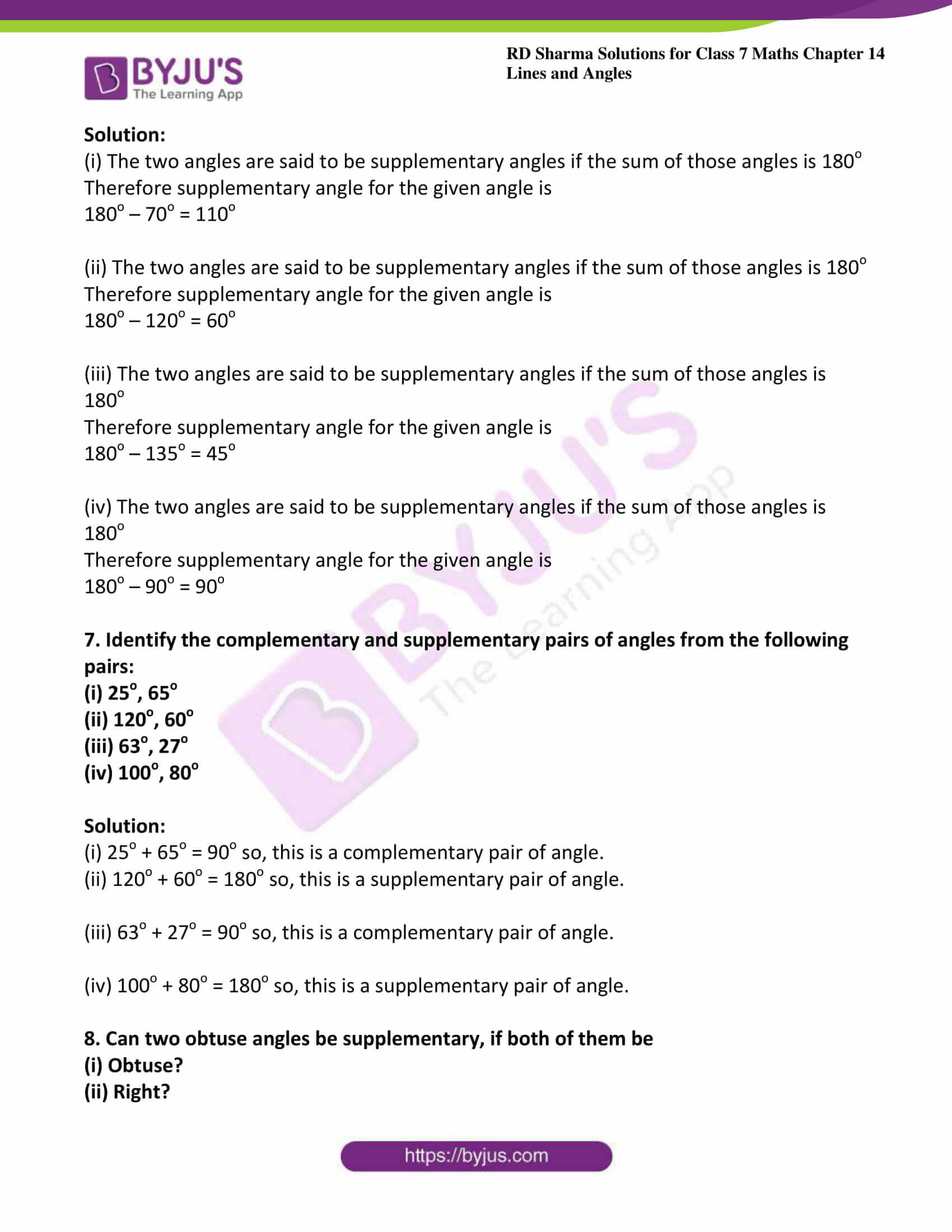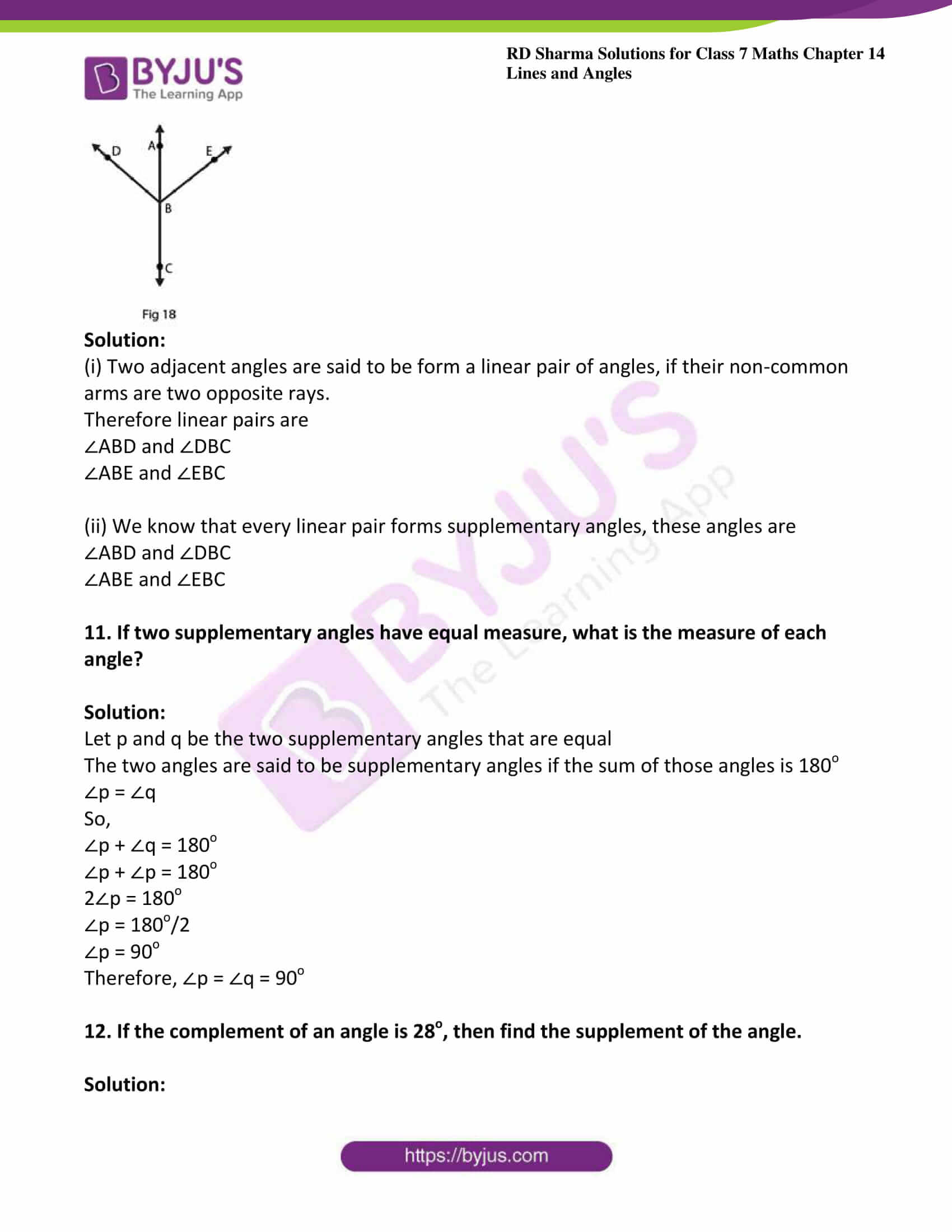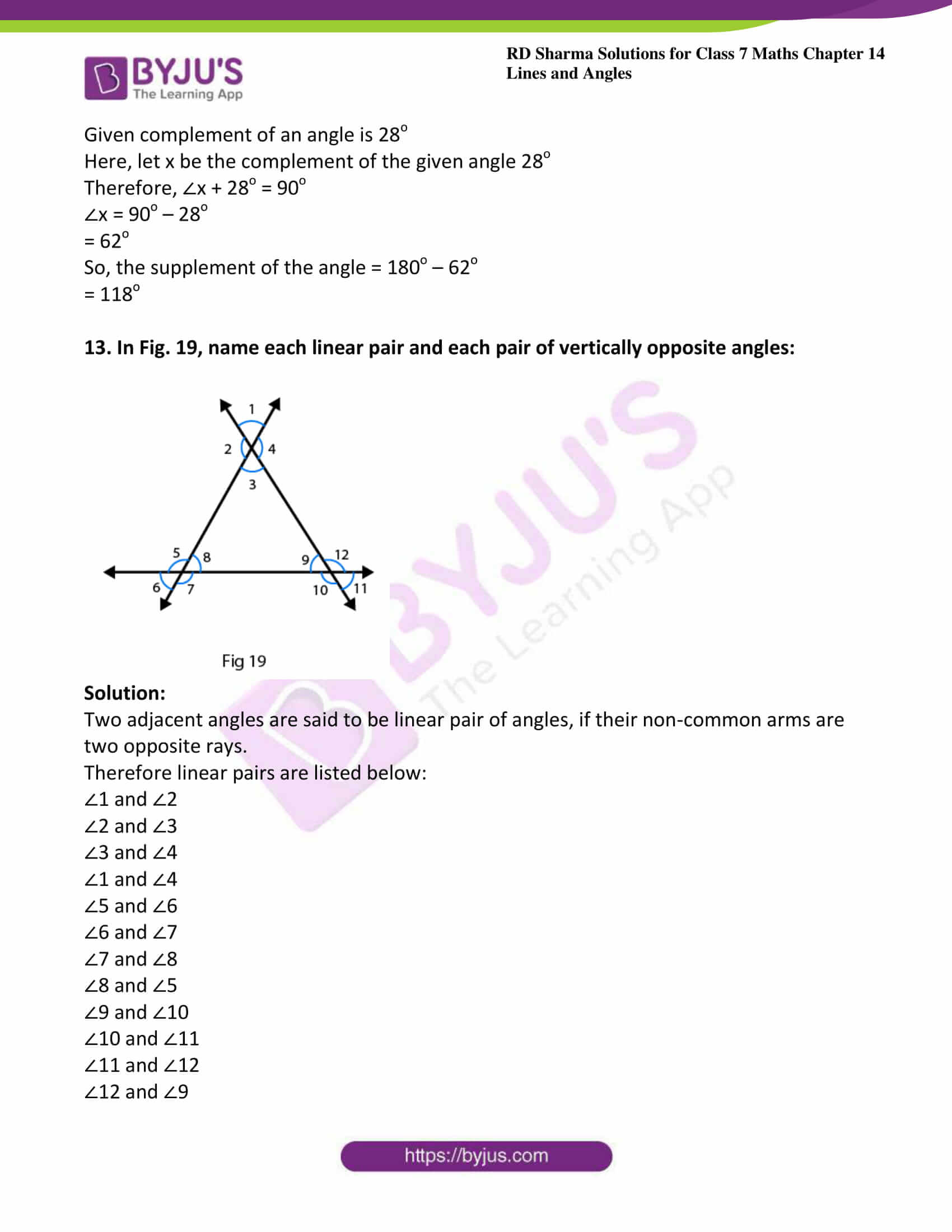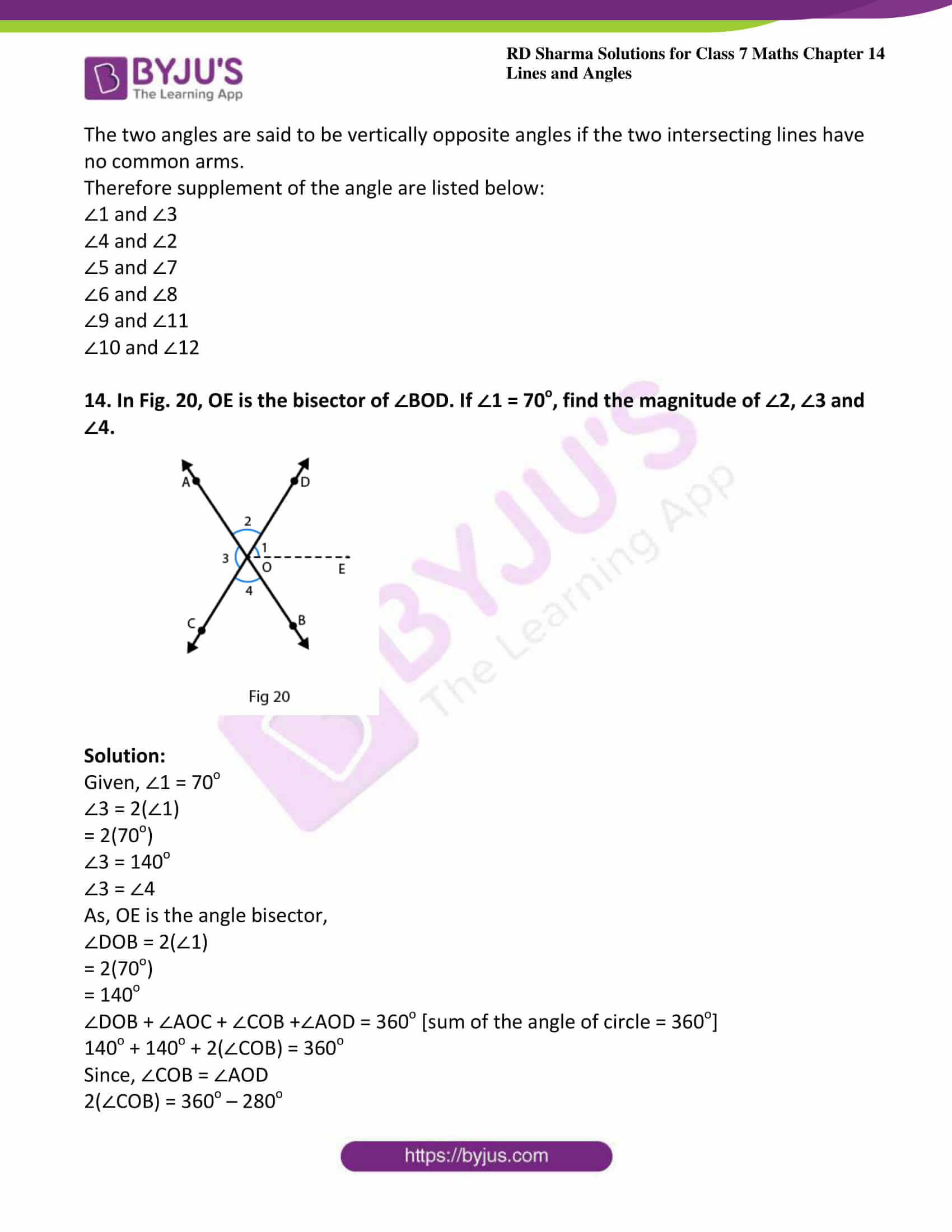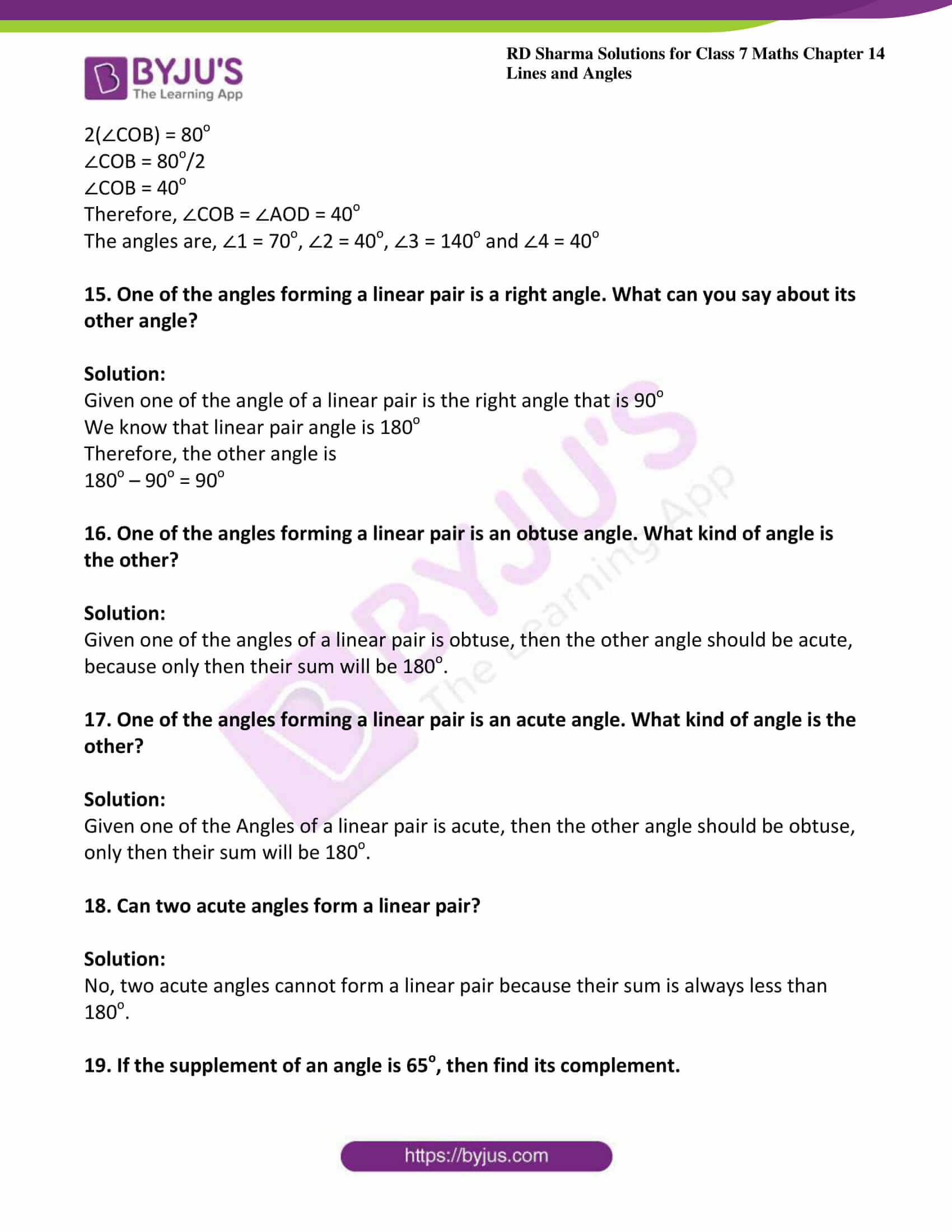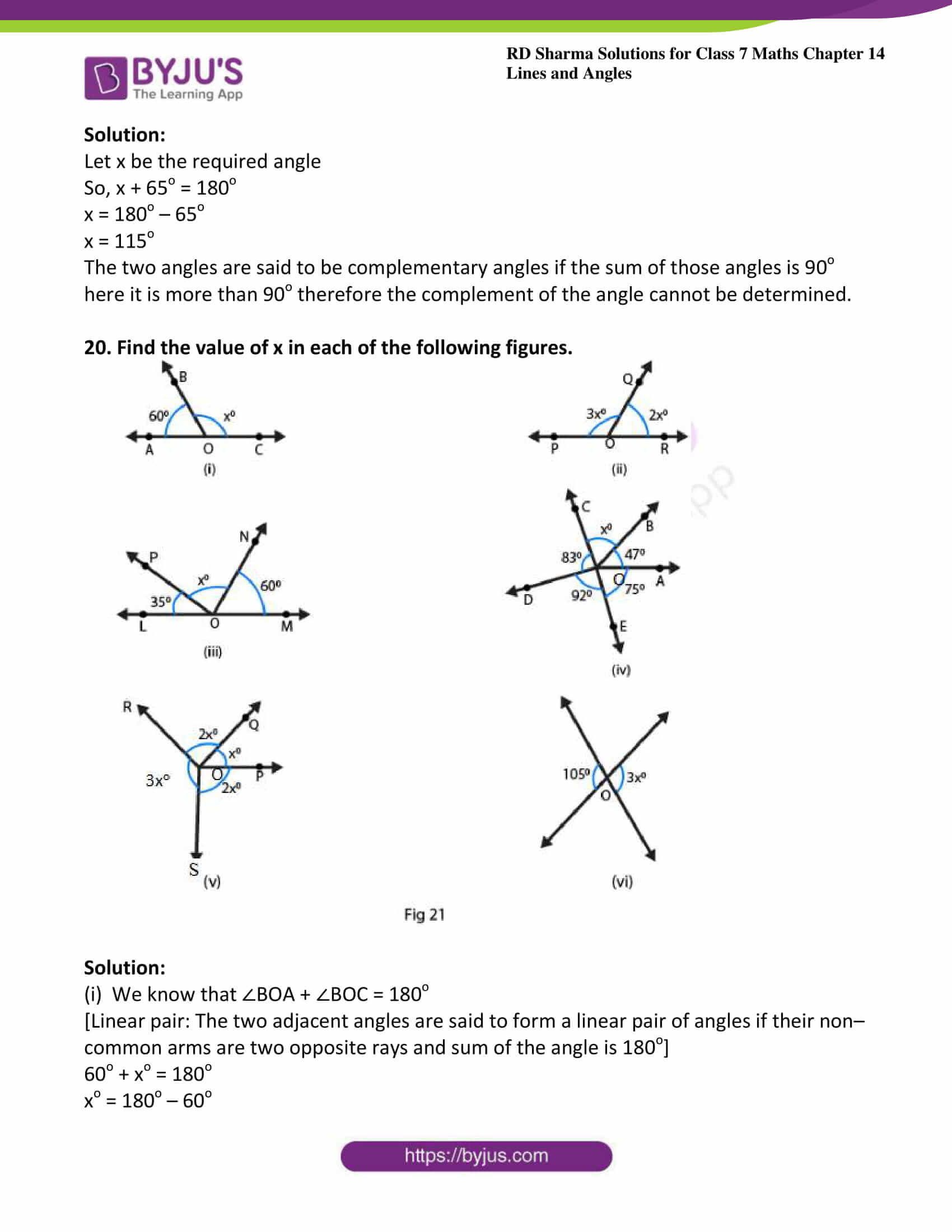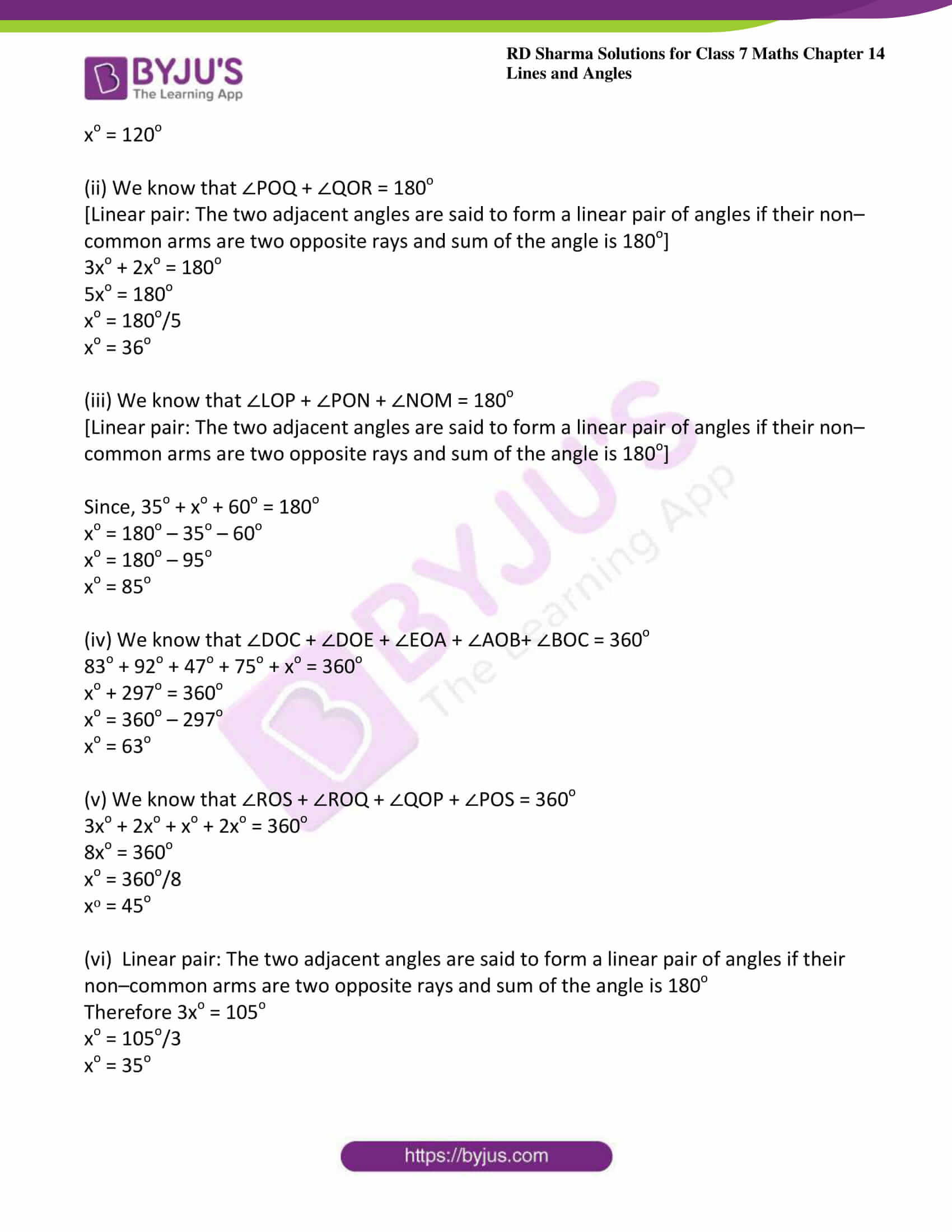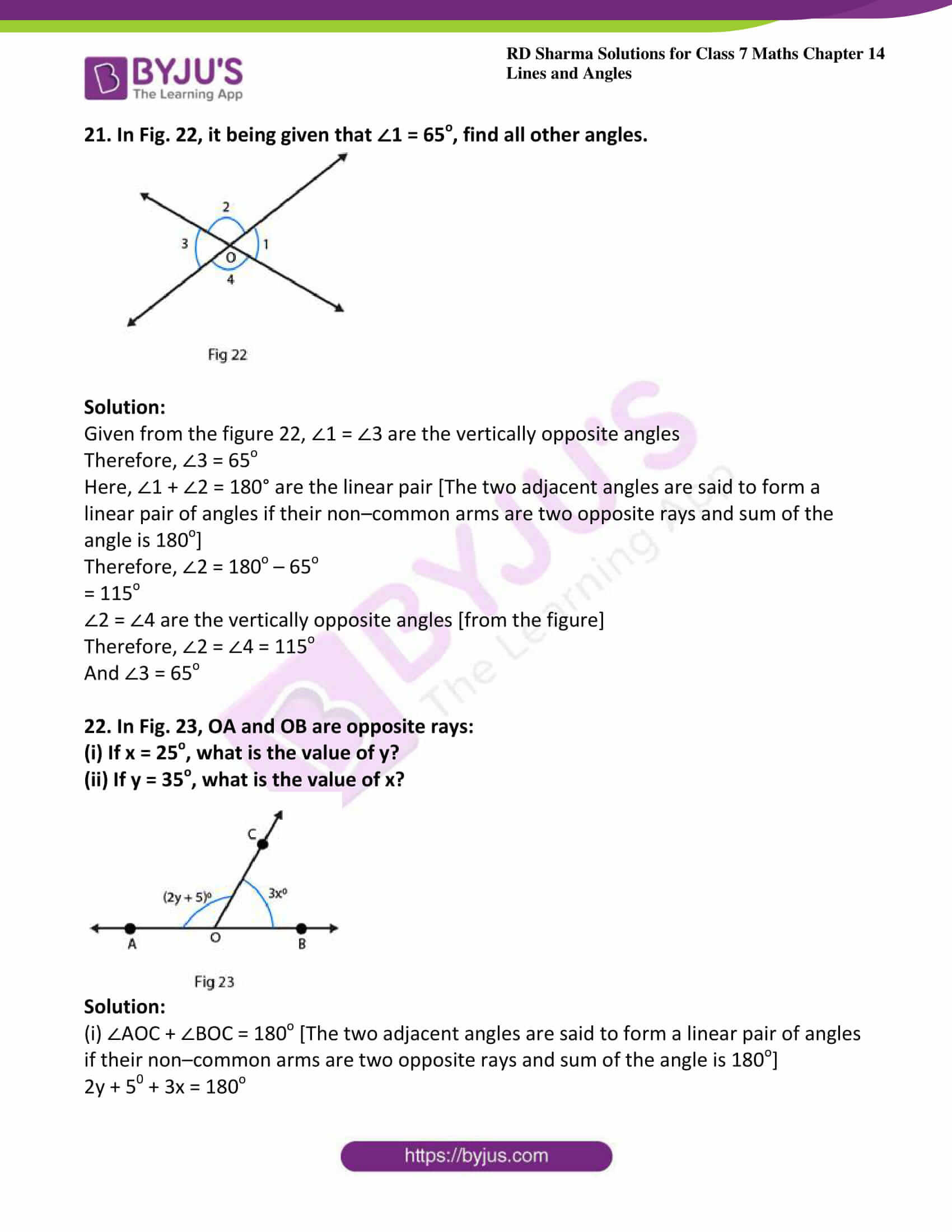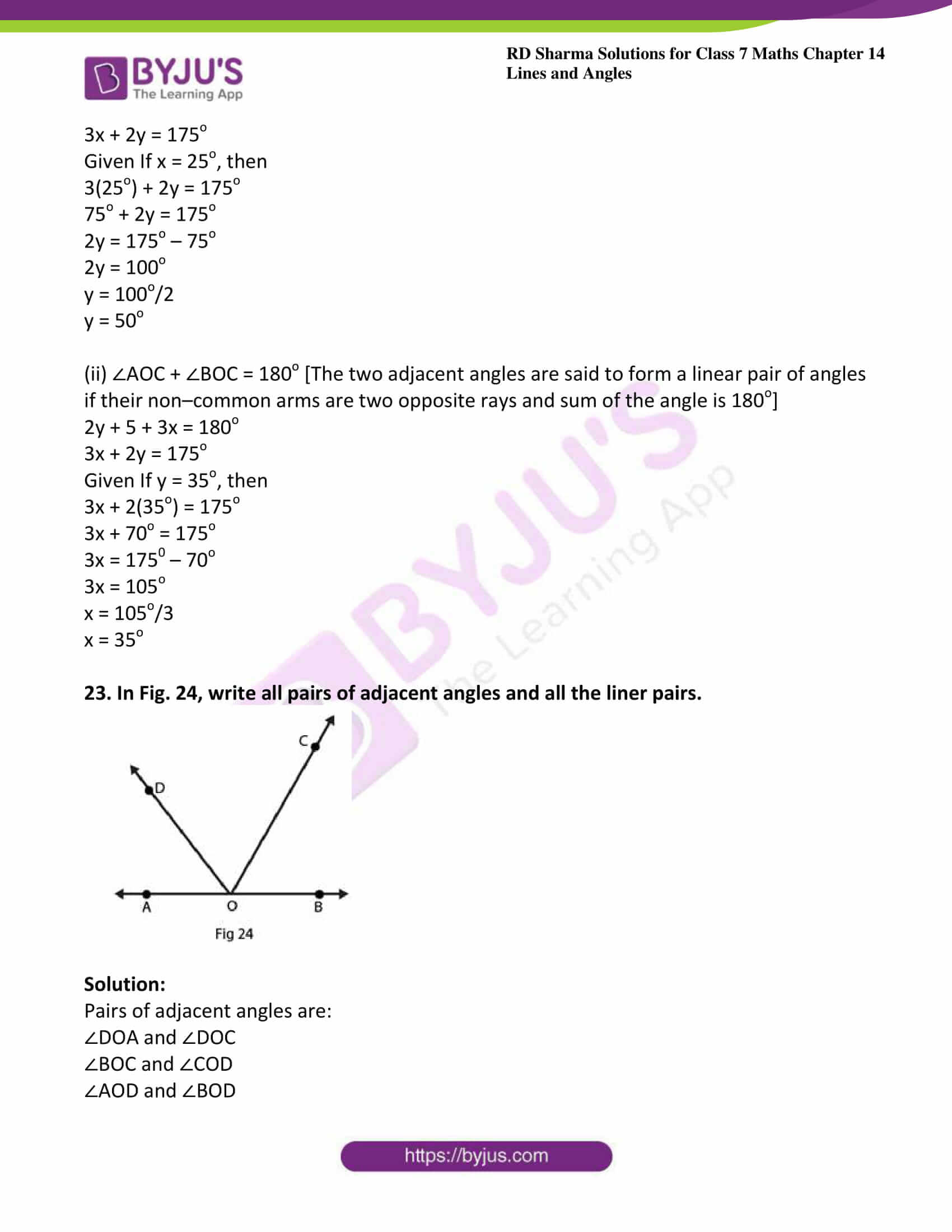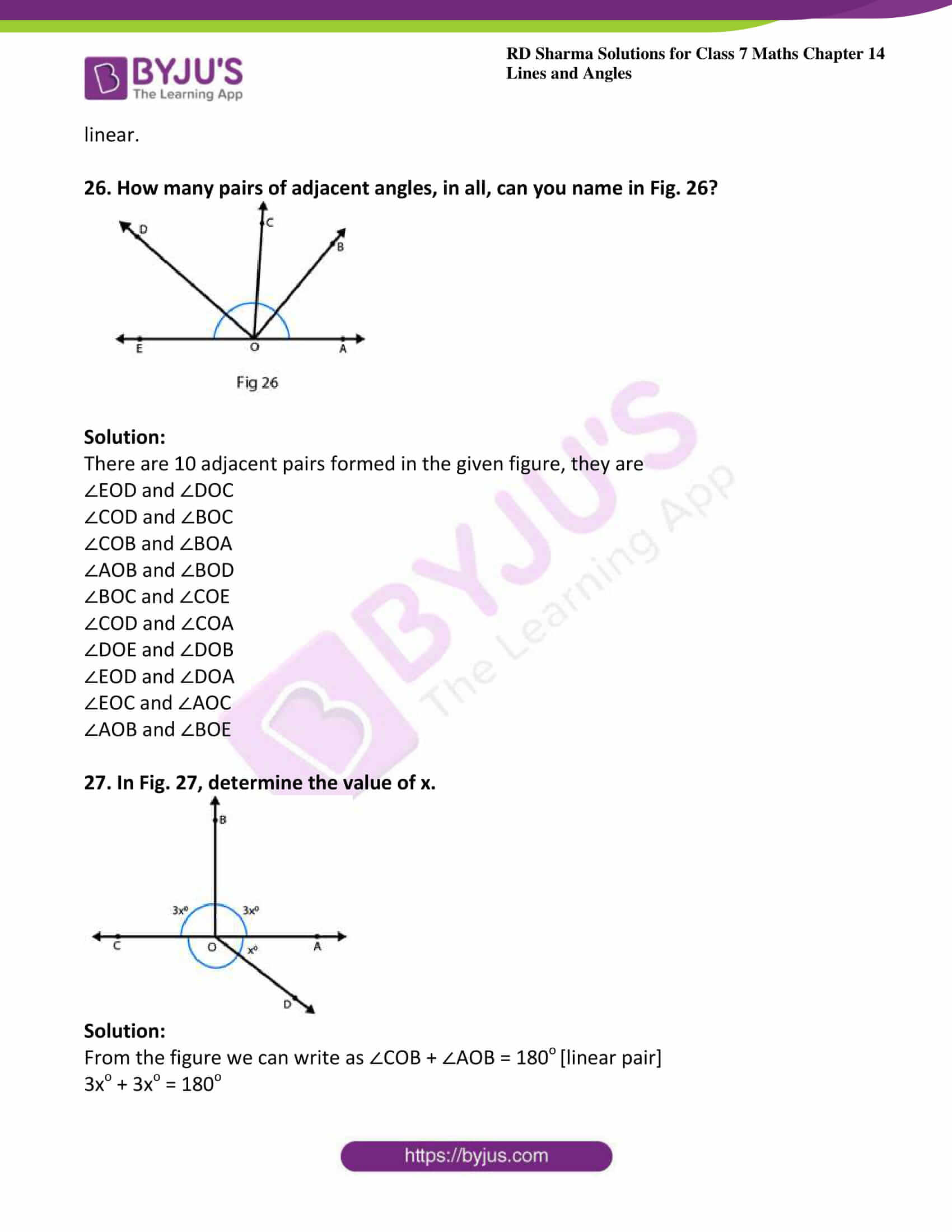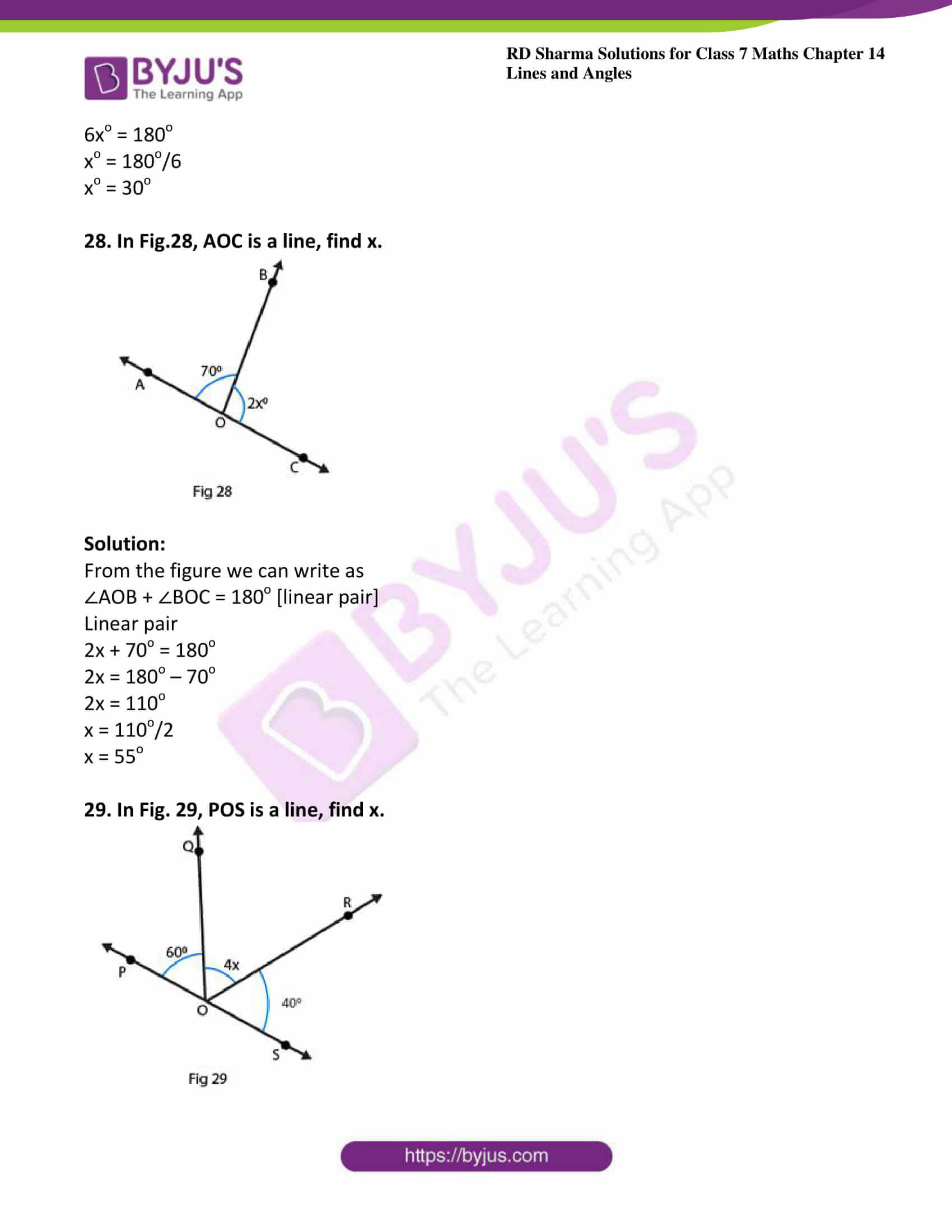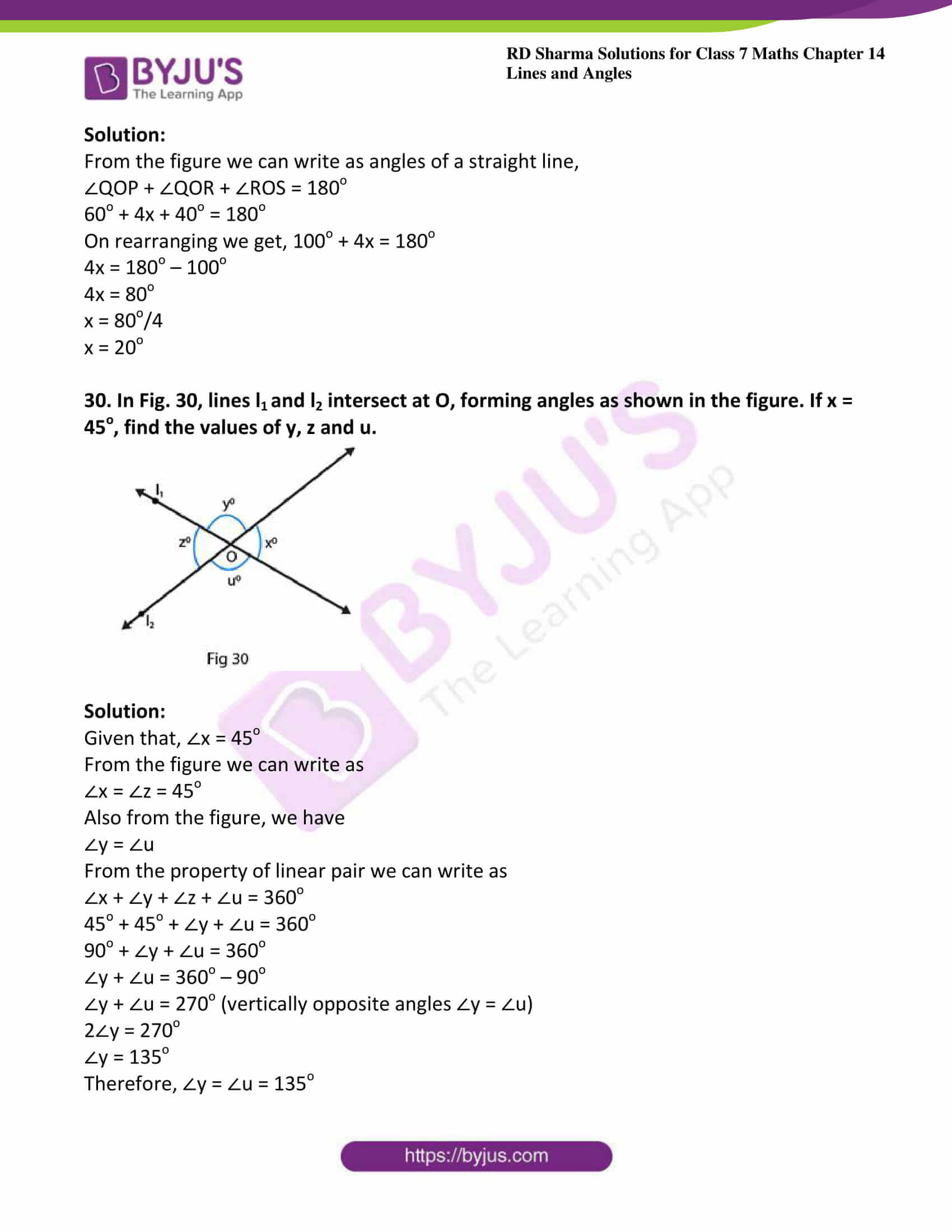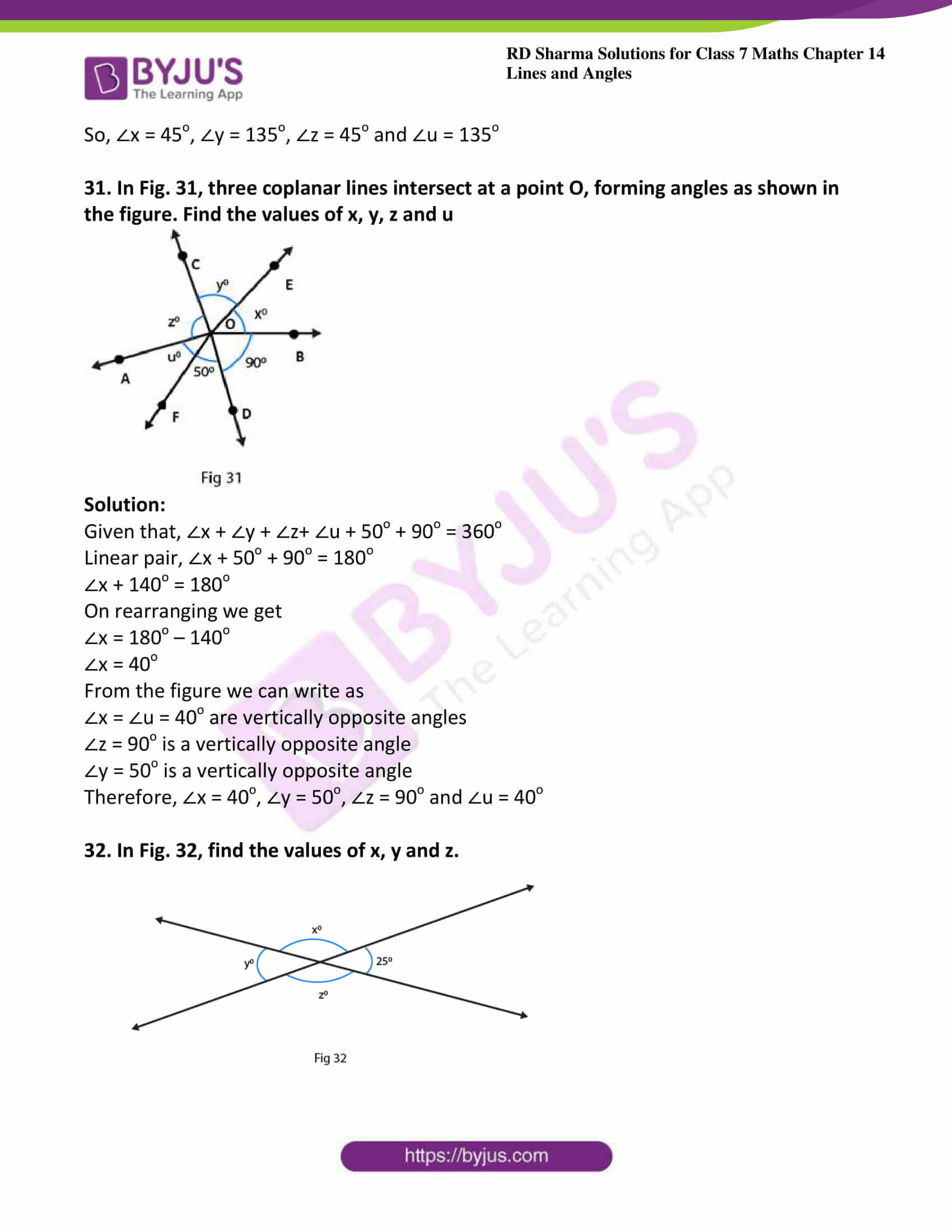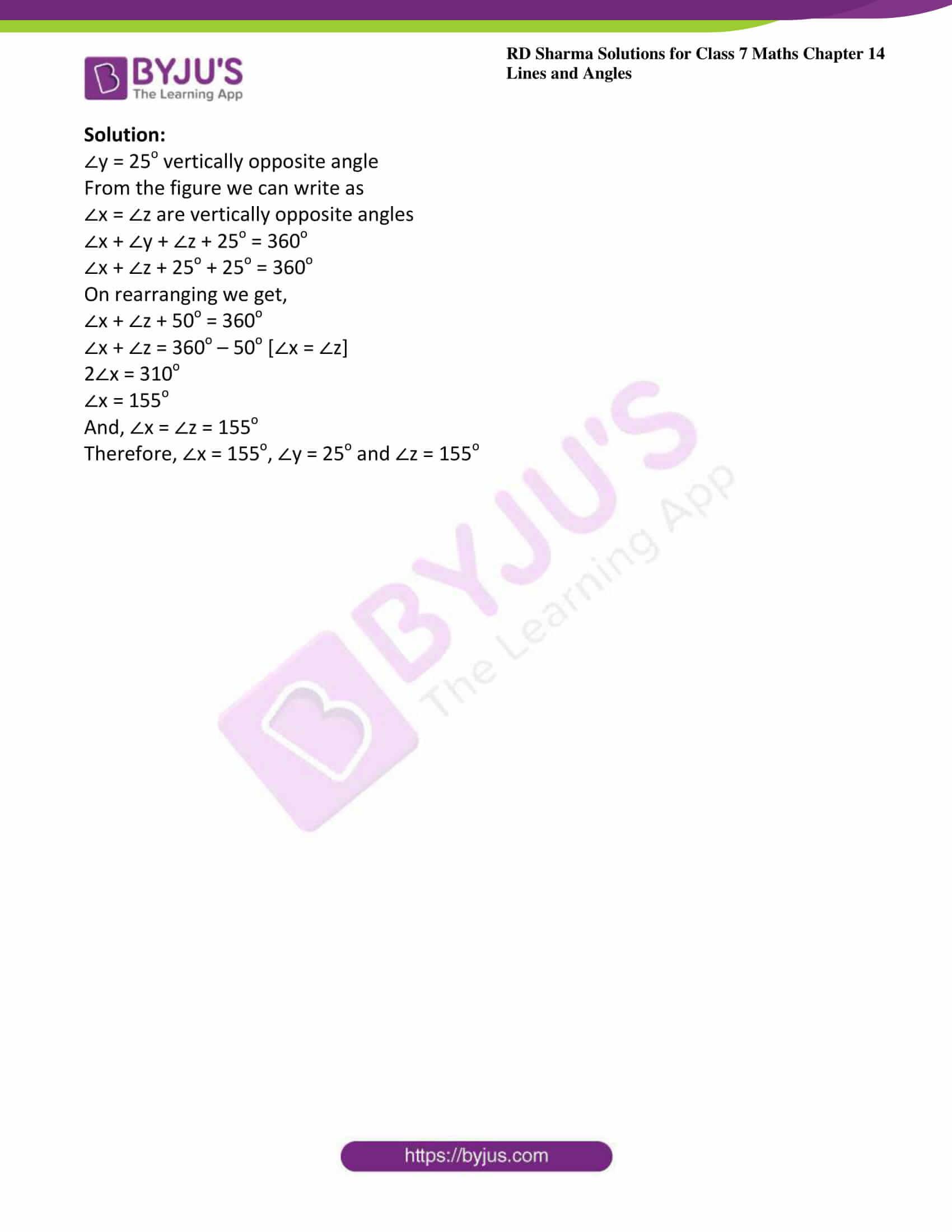### Access answers to Maths RD Sharma Solutions For Class 7 Chapter 14 – Lines And Angles Exercise 14.1

Exercise 14.1 Page No: 14.6

1. Write down each pair of adjacent angles shown in fig. 13.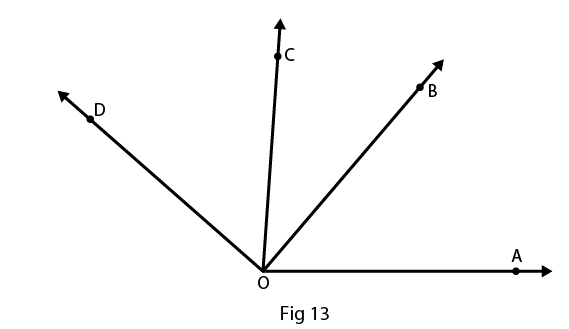Solution:

The angles that have common vertex and a common arm are known as adjacent angles

Therefore the adjacent angles in given figure are:

∠DOC and ∠BOC

∠COB and ∠BOA

2. In Fig. 14, name all the pairs of adjacent angles.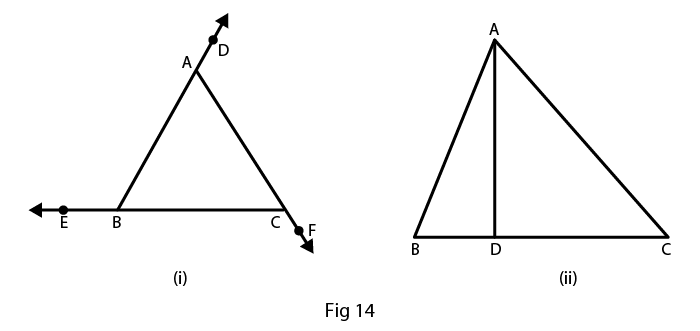Solution:

The angles that have common vertex and a common arm are known as adjacent angles.

In fig (i), the adjacent angles are

∠EBA and ∠ABC

∠ACB and ∠BCF

In fig (ii), the adjacent angles are

∠BDA and ∠CDA

3. In fig. 15, write down

(i) Each linear pair

(ii) Each pair of vertically opposite angles.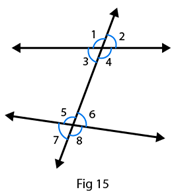Solution:

(i) The two adjacent angles are said to form a linear pair of angles if their non – common arms are two opposite rays.

∠1 and ∠3

∠1 and ∠2

∠4 and ∠3

∠4 and ∠2

∠5 and ∠6

∠5 and ∠7

∠6 and ∠8

∠7 and ∠8

(ii) The two angles formed by two intersecting lines and have no common arms are called vertically opposite angles.

∠1 and ∠4

∠2 and ∠3

∠5 and ∠8

∠6 and ∠7

4. Are the angles 1 and 2 given in Fig. 16 adjacent angles?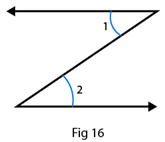Solution:

No, because they don’t have common vertex.

5. Find the complement of each of the following angles:

(i) 35o

(ii) 72o

(iii) 45o

(iv) 85o

Solution:

(i) The two angles are said to be complementary angles if the sum of those angles is 90o

Complementary angle for given angle is

90o – 35o = 55o

(ii) The two angles are said to be complementary angles if the sum of those angles is 90o

Complementary angle for given angle is

900 – 72o = 18o

(iii) The two angles are said to be complementary angles if the sum of those angles is 90o

Complementary angle for given angle is

90o – 45o = 45o

(iv) The two angles are said to be complementary angles if the sum of those angles is 90o

Complementary angle for given angle is

90o – 85o = 5o

6. Find the supplement of each of the following angles:

(i) 70o

(ii) 120o

(iii) 135o

(iv) 90o

Solution:

(i) The two angles are said to be supplementary angles if the sum of those angles is 180o

Therefore supplementary angle for the given angle is

180o – 70o = 110o

(ii) The two angles are said to be supplementary angles if the sum of those angles is 180o

Therefore supplementary angle for the given angle is

180o – 120o = 60o

(iii) The two angles are said to be supplementary angles if the sum of those angles is 180o

Therefore supplementary angle for the given angle is

180o – 135o = 45o

(iv) The two angles are said to be supplementary angles if the sum of those angles is 180o

Therefore supplementary angle for the given angle is

180o – 90o = 90o

7. Identify the complementary and supplementary pairs of angles from the following pairs:

(i) 25o, 65o

(ii) 120o, 60o

(iii) 63o, 27o

(iv) 100o, 80o

Solution:

(i) 25o + 65o = 90o so, this is a complementary pair of angle.

(ii) 120o + 60o = 180o so, this is a supplementary pair of angle.

(iii) 63o + 27o = 90o so, this is a complementary pair of angle.

(iv) 100o + 80o = 180o so, this is a supplementary pair of angle.

8. Can two obtuse angles be supplementary, if both of them be

(i) Obtuse?

(ii) Right?

(iii) Acute?

Solution:

(i) No, two obtuse angles cannot be supplementary

Because, the sum of two angles is greater than 90o so their sum will be greater than 180o

(ii) Yes, two right angles can be supplementary

Because, 90o + 90o = 180o

(iii) No, two acute angle cannot be supplementary

Because, the sum of two angles is less than 90o so their sum will also be less than 90o

9. Name the four pairs of supplementary angles shown in Fig.17.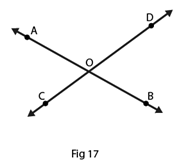Solution:

The two angles are said to be supplementary angles if the sum of those angles is 180o

The supplementary angles are

∠AOC and ∠COB

∠BOC and ∠DOB

∠BOD and ∠DOA

∠AOC and ∠DOA

10. In Fig. 18, A, B, C are collinear points and ∠DBA = ∠EBA.

(i) Name two linear pairs.

(ii) Name two pairs of supplementary angles.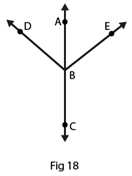Solution:

(i) Two adjacent angles are said to be form a linear pair of angles, if their non-common arms are two opposite rays.

Therefore linear pairs are

∠ABD and ∠DBC

∠ABE and ∠EBC

(ii) We know that every linear pair forms supplementary angles, these angles are

∠ABD and ∠DBC

∠ABE and ∠EBC

11. If two supplementary angles have equal measure, what is the measure of each angle?

Solution:

Let p and q be the two supplementary angles that are equal

The two angles are said to be supplementary angles if the sum of those angles is 180o

∠p = ∠q

So,

∠p + ∠q = 180o

∠p + ∠p = 180o

2∠p = 180o

∠p = 180o/2

∠p = 90o

Therefore, ∠p = ∠q = 90o

12. If the complement of an angle is 28o, then find the supplement of the angle.

Solution:

Given complement of an angle is 28o

Here, let x be the complement of the given angle 28o

Therefore, ∠x + 28o = 90o

∠x = 90o – 28o

= 62o

So, the supplement of the angle = 180o – 62o

= 118o

13. In Fig. 19, name each linear pair and each pair of vertically opposite angles: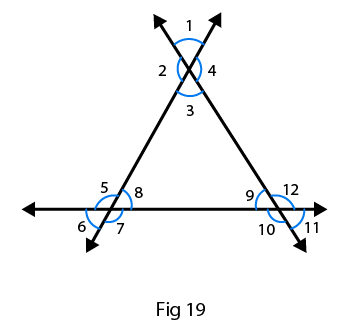Solution:

Two adjacent angles are said to be linear pair of angles, if their non-common arms are two opposite rays.

Therefore linear pairs are listed below:

∠1 and ∠2

∠2 and ∠3

∠3 and ∠4

∠1 and ∠4

∠5 and ∠6

∠6 and ∠7

∠7 and ∠8

∠8 and ∠5

∠9 and ∠10

∠10 and ∠11

∠11 and ∠12

∠12 and ∠9

The two angles are said to be vertically opposite angles if the two intersecting lines have no common arms.

Therefore supplement of the angle are listed below:

∠1 and ∠3

∠4 and ∠2

∠5 and ∠7

∠6 and ∠8

∠9 and ∠11

∠10 and ∠12

14. In Fig. 20, OE is the bisector of ∠BOD. If ∠1 = 70o, find the magnitude of ∠2, ∠3 and ∠4.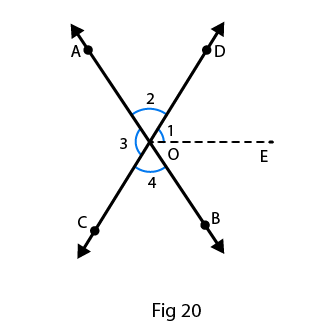Solution:

Given, ∠1 = 70o

∠3 = 2(∠1)

= 2(70o)

∠3 = 140o

∠3 = ∠4

As, OE is the angle bisector,

∠DOB = 2(∠1)

= 2(70o)

= 140o

∠DOB + ∠AOC + ∠COB +∠AOD = 360o [sum of the angle of circle = 360o]

140o + 140o + 2(∠COB) = 360o

Since, ∠COB = ∠AOD

2(∠COB) = 360o – 280o

2(∠COB) = 80o

∠COB = 80o/2

∠COB = 40o

Therefore, ∠COB = ∠AOD = 40o

The angles are, ∠1 = 70o, ∠2 = 40o, ∠3 = 140o and ∠4 = 40o

15. One of the angles forming a linear pair is a right angle. What can you say about its other angle?

Solution:

Given one of the angle of a linear pair is the right angle that is 90o

We know that linear pair angle is 180o

Therefore, the other angle is

180o – 90o = 90o

16. One of the angles forming a linear pair is an obtuse angle. What kind of angle is the other?

Solution:

Given one of the angles of a linear pair is obtuse, then the other angle should be acute, because only then their sum will be 180o.

17. One of the angles forming a linear pair is an acute angle. What kind of angle is the other?

Solution:

Given one of the Angles of a linear pair is acute, then the other angle should be obtuse, only then their sum will be 180o.

18. Can two acute angles form a linear pair?

Solution:

No, two acute angles cannot form a linear pair because their sum is always less than 180o.

19. If the supplement of an angle is 65o, then find its complement.

Solution:

Let x be the required angle

So, x + 65o = 180o

x = 180o – 65o

x = 115o

The two angles are said to be complementary angles if the sum of those angles is 90o here it is more than 90o therefore the complement of the angle cannot be determined.

20. Find the value of x in each of the following figures.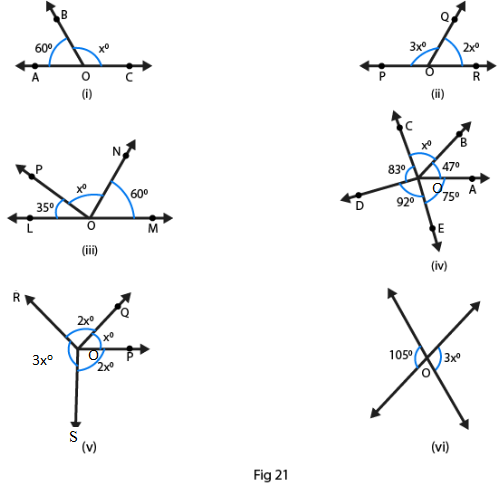Solution:

(i)  We know that ∠BOA + ∠BOC = 180o

[Linear pair: The two adjacent angles are said to form a linear pair of angles if their non–common arms are two opposite rays and sum of the angle is 180o]

60o + xo = 180o

xo = 180o – 60o

xo = 120o

(ii) We know that ∠POQ + ∠QOR = 180o

[Linear pair: The two adjacent angles are said to form a linear pair of angles if their non–common arms are two opposite rays and sum of the angle is 180o]

3xo + 2xo = 180o

5xo = 180o

xo = 180o/5

xo = 36o

(iii) We know that ∠LOP + ∠PON + ∠NOM = 180o

[Linear pair: The two adjacent angles are said to form a linear pair of angles if their non–common arms are two opposite rays and sum of the angle is 180o]

Since, 35o + xo + 60o = 180o

xo = 180o – 35o – 60o

xo = 180o – 95o

xo = 85o

(iv) We know that ∠DOC + ∠DOE + ∠EOA + ∠AOB+ ∠BOC = 360o

83o + 92o + 47o + 75o + xo = 360o

xo + 297o = 360o

xo = 360o – 297o

xo = 63o

(v) We know that ∠ROS + ∠ROQ + ∠QOP + ∠POS = 360o

3xo + 2xo + xo + 2xo = 360o

8xo = 360o

xo = 360o/8

xo = 45o

(vi)  Linear pair: The two adjacent angles are said to form a linear pair of angles if their non–common arms are two opposite rays and sum of the angle is 180o

Therefore 3xo = 105o

xo = 105o/3

xo = 35o

21. In Fig. 22, it being given that ∠1 = 65o, find all other angles.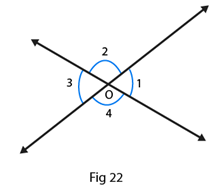Solution:

Given from the figure 22, ∠1 = ∠3 are the vertically opposite angles

Therefore, ∠3 = 65o

Here, ∠1 + ∠2 = 180° are the linear pair [The two adjacent angles are said to form a linear pair of angles if their non–common arms are two opposite rays and sum of the angle is 180o]

Therefore, ∠2 = 180o – 65o

= 115o

∠2 = ∠4 are the vertically opposite angles [from the figure]

Therefore, ∠2 = ∠4 = 115o

And ∠3 = 65o

22. In Fig. 23, OA and OB are opposite rays:

(i) If x = 25o, what is the value of y?

(ii) If y = 35o, what is the value of x?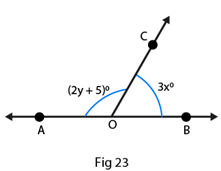Solution:

(i) ∠AOC + ∠BOC = 180o [The two adjacent angles are said to form a linear pair of angles if their non–common arms are two opposite rays and sum of the angle is 180o]

2y + 50 + 3x = 180o

3x + 2y = 175o

Given If x = 25o, then

3(25o) + 2y = 175o

75o + 2y = 175o

2y = 175o – 75o

2y = 100o

y = 100o/2

y = 50o

(ii) ∠AOC + ∠BOC = 180o [The two adjacent angles are said to form a linear pair of angles if their non–common arms are two opposite rays and sum of the angle is 180o]

2y + 5 + 3x = 180o

3x + 2y = 175o

Given If y = 35o, then

3x + 2(35o) = 175o

3x + 70o = 175o

3x = 1750 – 70o

3x = 105o

x = 105o/3

x = 35o

23. In Fig. 24, write all pairs of adjacent angles and all the liner pairs.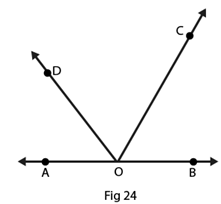Solution:

∠DOA and ∠DOC

∠BOC and ∠COD

∠AOD and ∠BOD

∠AOC and ∠BOC

Linear pairs: [The two adjacent angles are said to form a linear pair of angles if their non–common arms are two opposite rays and sum of the angle is 180o]

∠AOD and ∠BOD

∠AOC and ∠BOC

24. In Fig. 25, find ∠x. Further find ∠BOC, ∠COD and ∠AOD.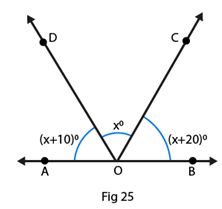Solution:

(x + 10)o + xo + (x + 20)o = 180o[linear pair]

On rearranging we get

3xo + 30o = 180o

3xo = 180o – 30o

3xo = 150o

xo = 150o/3

xo = 50o

Also given that

∠BOC = (x + 20)o

= (50 + 20)o

= 70o

∠COD = 50o

∠AOD = (x + 10)o

= (50 + 10)o

= 60o

25. How many pairs of adjacent angles are formed when two lines intersect in a point?

Solution:

If the two lines intersect at a point, then four adjacent pairs are formed and those are linear.

26. How many pairs of adjacent angles, in all, can you name in Fig. 26?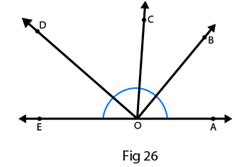Solution:

There are 10 adjacent pairs formed in the given figure, they are

∠EOD and ∠DOC

∠COD and ∠BOC

∠COB and ∠BOA

∠AOB and ∠BOD

∠BOC and ∠COE

∠COD and ∠COA

∠DOE and ∠DOB

∠EOD and ∠DOA

∠EOC and ∠AOC

∠AOB and ∠BOE

27. In Fig. 27, determine the value of x.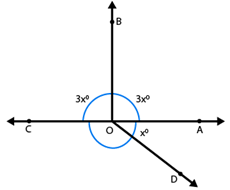Solution:

From the figure we can write as ∠COB + ∠AOB = 180o [linear pair]

3xo + 3xo = 180o

6xo = 180o

xo = 180o/6

xo = 30o

28. In Fig.28, AOC is a line, find x.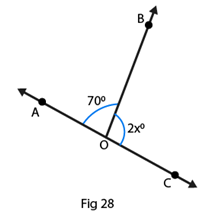Solution:

From the figure we can write as

∠AOB + ∠BOC = 180o [linear pair]

Linear pair

2x + 70o = 180o

2x = 180o – 70o

2x = 110o

x = 110o/2

x = 55o

29. In Fig. 29, POS is a line, find x.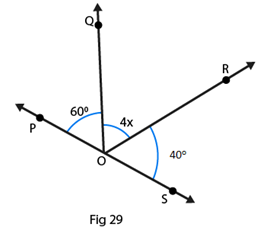Solution:

From the figure we can write as angles of a straight line,

∠QOP + ∠QOR + ∠ROS = 180o

60o + 4x + 40o = 180o

On rearranging we get, 100o + 4x = 180o

4x = 180o – 100o

4x = 80o

x = 80o/4

x = 20o

30. In Fig. 30, lines l1 and l2 intersect at O, forming angles as shown in the figure. If x = 45o, find the values of y, z and u.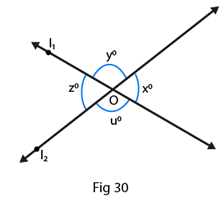Solution:

Given that, ∠x = 45o

From the figure we can write as

∠x = ∠z = 45o

Also from the figure, we have

∠y = ∠u

From the property of linear pair we can write as

∠x + ∠y + ∠z + ∠u = 360o

45o + 45o + ∠y + ∠u = 360o

90o + ∠y + ∠u = 360o

∠y + ∠u = 360o – 90o

∠y + ∠u = 270o (vertically opposite angles ∠y = ∠u)

2∠y = 270o

∠y = 135o

Therefore, ∠y = ∠u = 135o

So, ∠x = 45o, ∠y = 135o, ∠z = 45o and ∠u = 135o

31. In Fig. 31, three coplanar lines intersect at a point O, forming angles as shown in the figure. Find the values of x, y, z and u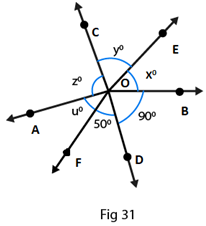Solution:

Given that, ∠x + ∠y + ∠z+ ∠u + 50o + 90o = 360o

Linear pair, ∠x + 50o + 90o = 180o

∠x + 140o = 180o

On rearranging we get

∠x = 180o – 140o

∠x = 40o

From the figure we can write as

∠x = ∠u = 40o are vertically opposite angles

∠z = 90o is a vertically opposite angle

∠y = 50o is a vertically opposite angle

Therefore, ∠x = 40o, ∠y = 50o, ∠z = 90o and ∠u = 40o

32. In Fig. 32, find the values of x, y and z.Solution:

∠y = 25o vertically opposite angle

From the figure we can write as

∠x = ∠z are vertically opposite angles

∠x + ∠y + ∠z + 25o = 360o

∠x + ∠z + 25o + 25o = 360o

On rearranging we get,

∠x + ∠z + 50o = 360o

∠x + ∠z = 360o – 50o [∠x = ∠z]

2∠x = 310o

∠x = 155o

And, ∠x = ∠z = 155o

Therefore, ∠x = 155o, ∠y = 25o and ∠z = 155o